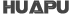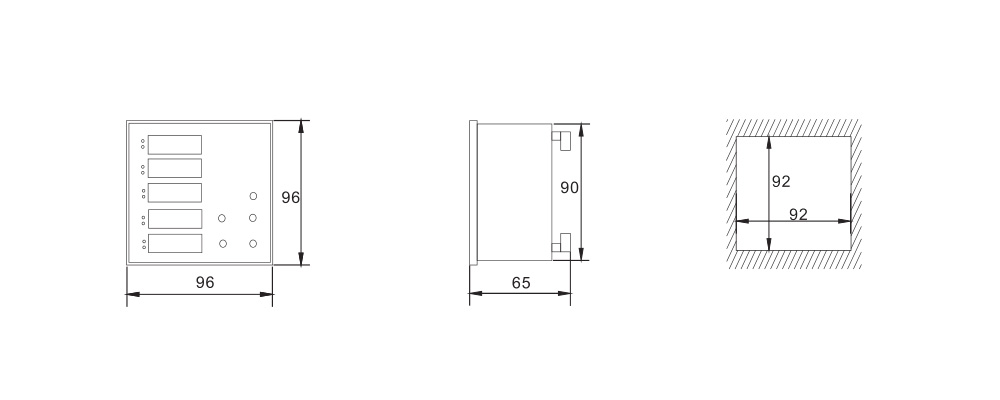## Digital display Demand Indication Multi-functional Electric MeterProduct Profile
Function FeatureThis meter adopts the five-row LED display equipped with the way of status indicators take turnsto show to real-time measure and display the various electric parameters of three-phase electric circuits, such as voltage, current, frequ

# Function Feature

This meter adopts the five-row LED display equipped with the way of status indicators take turnsto show to real-time measure and display the various electric parameters of three-phase electric circuits, such as voltage, current, frequency, power, power factor, four quadrant energy, and soon. It also provides the functions of demand indication, maximum value record, minimum value record, and so on.

Short shell design, compact, economical and practical.
True RMS measurement, frequency and waveform change will not influence the accuracy of measurement.
It can display directly multiple electric parameters at the same time.
PT and CT ratio can be set.
Statistics of extreme values and demand indication, and the demand slip time can be set.
Buckle mounting way make the installation efficient, firm and reliable.
It can extend one channel RS485 communication interface, MODBUS-RTU communication protocol.

# Measuring and Display

Phase-zero voltage(VL_N)
Phase-phase voltage(VL_L)
Phase-current(A)
Total current(∑A)
Active power of each phase(W)
Reactive power of each phase(var)
Apparent power of each phase(VA)
Power factor of each phase(cosφ)
Active energy(Wh)
Reactive energy(varh)
Total harmonic distortion factor of
each phase voltage(THD%-U)

Total harmonic distortion factor of each phase current(THD%-1)Frequency(Hz)
Total active power(∑W)
Total reactive power(∑var)
Total apparent power(∑VA)
Total power factor(∑cosφ)
Each phase current,maximum value of total power(H)
Each phase current,minimum value of total power(L)
Each phase power,demand value of total power(M)

# Basic Parameter

Input specification:3XAC 220V/380V5A,3XAC 57.5V/100V 5A
Frequency range:40~65Hz
Power consumption of input loop:<1VA/phase
Resolving ability: for voltage, maximum is 0.1V; for current, maximum is 0.001A; decimal point will shit automatically
Measuring accuracy: for voltage, current and power, is ±(0.5% FS); for frequency, is ±0.1Hz; for power factor, is ±0.01 PF; for active energy, is class 1.0; for reactive energy, is class 2.0
RS485 communication: defaulted as no, it can be extended as one channel and adopts the MODBUS-RTU protocal
RS485 communication: defaulted as no, it can be extended as one channel and adopts the MODBUS-RTU protocal
Auxiliary power supply: AC/DC80~260V
Power consumption of power supply:<5VA
Working environment: temperature is -10～+50□, humidity<85% RH, without any corrosive gas
Safety withstand voltage: using the AC 2kV withstand voltage test to measure between the input terminal and the power supply terminal

# Contour and Installation Size(unit:mm)# Typical Connection